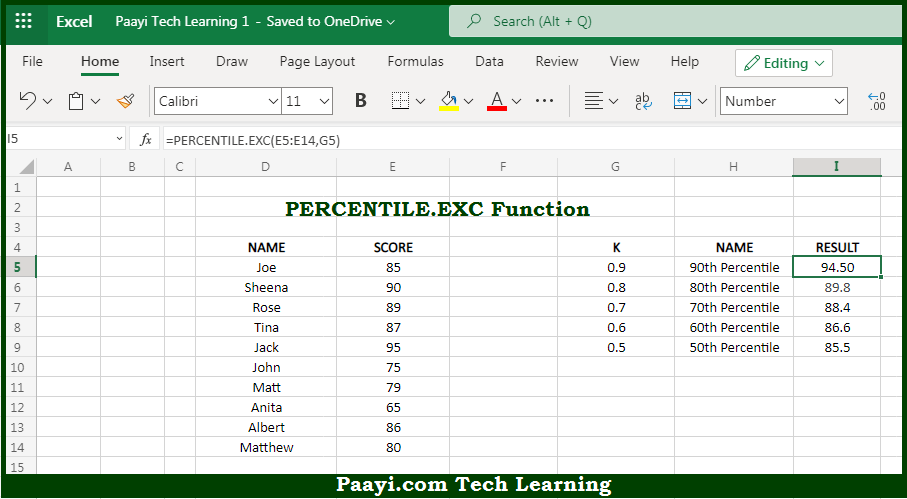# Learn How to Use Microsoft Excel PERCENTILE.EXC Function

Written by | 0 Comments | 523 Views

In this article, you will learn how to use Microsoft Excel PERCENTILE.EXC function and its prime function in Microsoft Excel. You will also get to know Microsoft Excel PERCENTILE.EXC function return value and syntax with the help of some examples.

Microsoft Excel PERCENTILE.EXC Function

The main purpose of Microsoft Excel PERCENTILE.EXC function is to get the kth percentile. That implies, with the help of PERCENTILE.EXC function you can able to calculates the "kth percentile" for the given set of data, where k is 0 to 1. It should be noted that percentile is a value below which a given percentage of values in a data set fall. You can also use PERCENTILE.EXC to determine the 90th percentile, the 80th percentile, and so on. So, with the help of PERCENTILE.EXC function, you can able to get the kth percentile.

Return Value of PERCENTILE.EXC Function

The return value will be the calculated percentile for "k".

Syntax of PERCENTILE.EXC Function

=PERCENTILE.EXC(array, k)

Where the arguments:

• array: This is the data value array.
• k: This is the value between 0 and 1 representing the kth percentile.

## How to Use Microsoft Excel PERCENTILE.EXC Function?So we know that, Microsoft Excel PERCENTILE.EXC function you can able to get the kth percentile. That implies, with the help of PERCENTILE.EXC function you can able to calculates the "kth percentile" for the given set of data, where k is 0 to 1. It should be noted that percentile is a value below which a given percentage of values in a data set fall. You can also use PERCENTILE.EXC to determine the 90th percentile, the 80th percentile, and so on. So, with the help of PERCENTILE.EXC function, you can able to get the kth percentile.